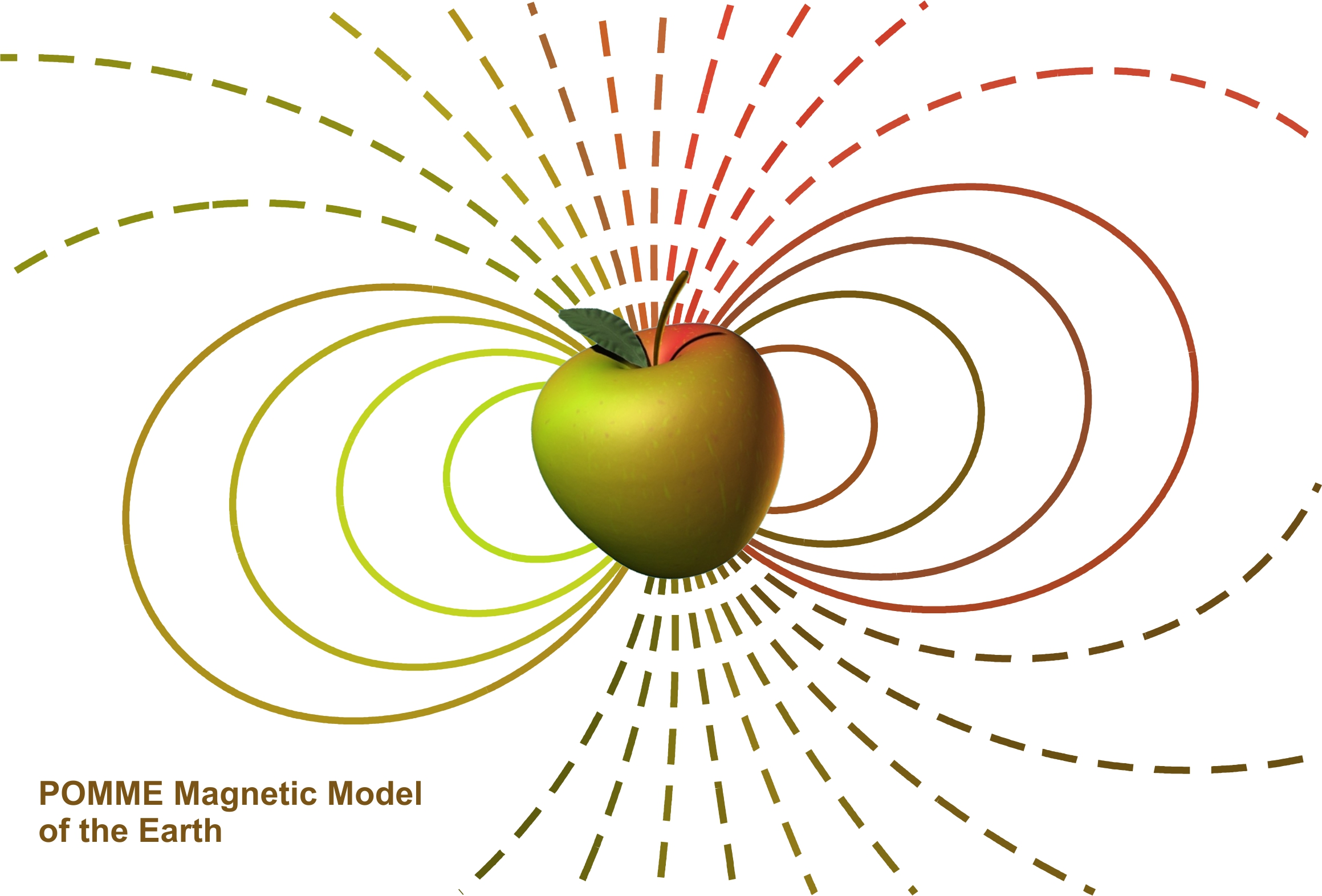## POMME-7 Magnetic Model of the Earth

POMME is a scientific main field model representing the geomagnetic field in the region from the Earth's surface to an altitude of a couple of thousand kilometers. The time variations of the internal field are given by a piece-wise linear representation of the spherical harmonic (Gauss) coefficients of the magnetic potential. Pomme-7 was produced from CHAMP satellite vector magnetic measurements from July 2000 up to September 2010 and Oersted satellite total field measurements from January 2010 to October 2011. It has the same parametrization of the magnetospheric field as POMME-6.

The model is more accurate if the present state of the magnetosphere is provided as an input in the form of magnetic indices. The magnetospheric ring current is tracked by Est/Ist (the default values for Est and Ist are both 0.0). The Interplanetary magnetic field and F10.7 index are available from OMNIWEB (default IMF-BY = 0.0). The merging electric field Em is a parameter derived from the solar wind measurements by: Em (in mV/m) = V * BT * sin(beta/2.0) * sin(beta/2.0) / 1000, where V is the solar wind flow speed in km/s, BT is the strength of the interplanetary magnetic field perpendicular to the Sun-Earth axis and beta is the clock angle acos(Bz/BT). A default value of 0.5 mV/m is to be used if the value of Em is not known. The model uses the 81-day average value of the F10.7 solar flux, 20 months prior to the given date, to track changes in the ring current base line with solar cycle progression (F10.7 default value is 120.0).SH coefficients